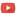KE8QZC | SFW | TSWSyllabus (1PM Section J): [pdf] [tex]
Syllabus (2PM Section H): [pdf] [tex]
Student calendar

Quizzes
Quiz 1 [pdf] [tex] | Quiz 1 (solution) [pdf] [tex]
Quiz 2 [pdf] [tex] | Quiz 2 (solution) [pdf] [tex]
Quiz 3 [pdf] [tex] | Quiz 3 (solution) [pdf] [tex]
Quiz 4 [pdf] [tex] | Quiz 4 (solution) [pdf] [tex]
Quiz 5 [pdf] [tex] | Quiz 5 (solution) [pdf] [tex]
Quiz 6 [pdf] [tex] | Quiz 6 (solution) [pdf] [tex]
Quiz 7 [pdf] [tex] | Quiz 7 (solution) [pdf] [tex]
Quiz 8 (do old exams)
Quiz 9 (do old exams)
Quiz 10 (do old exams)
Quiz 11 (do old exams)

Area of a cone with base $R$ and height $h$